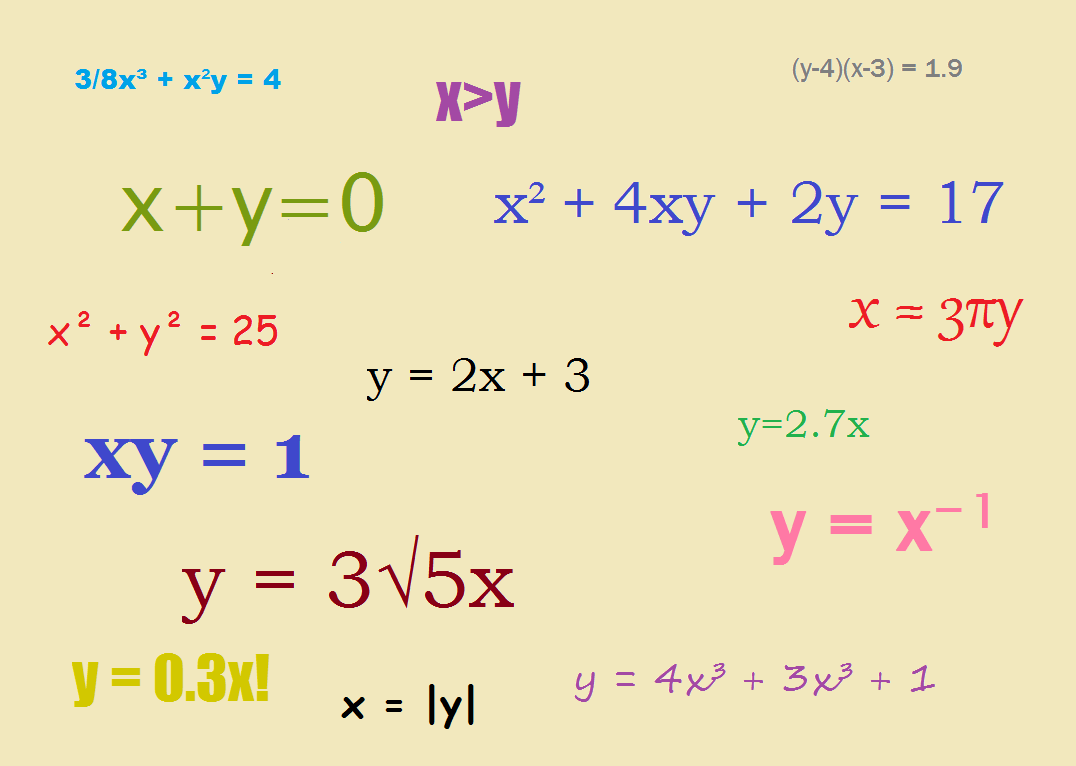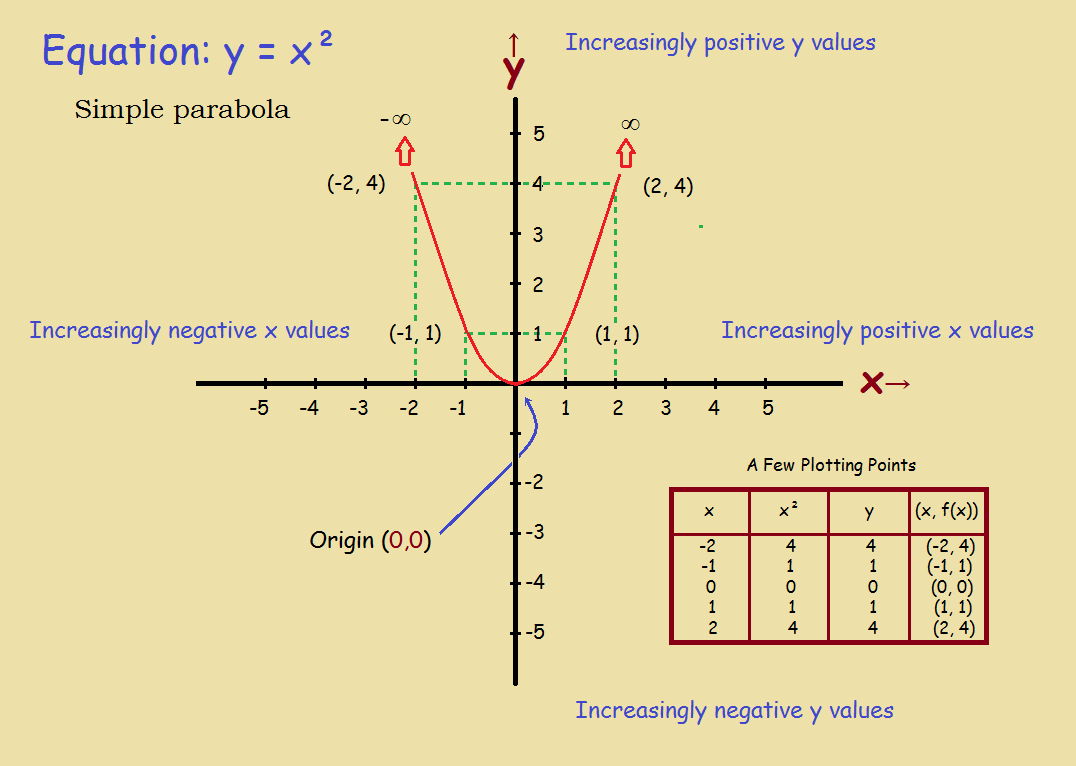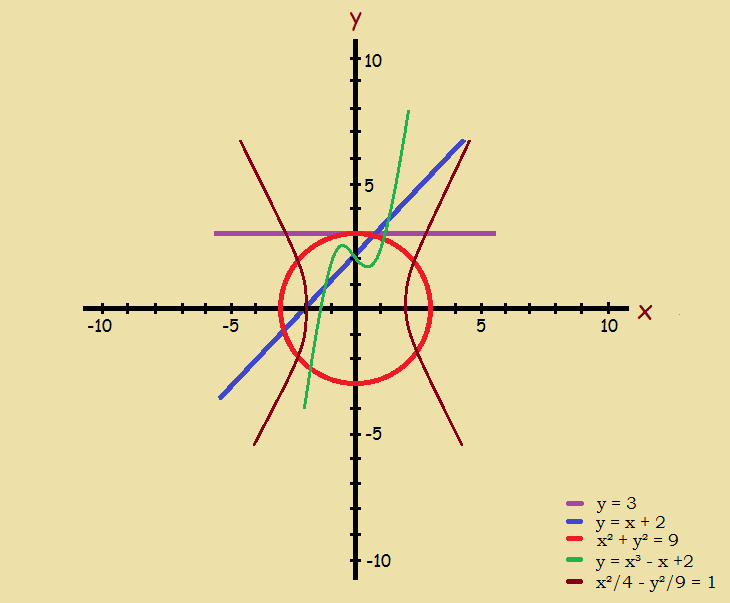Simple Algebra II Graph Symmetries Discussion and ExamplesTypical functions in two variables. College preparatory classes in high school often include Algebra and Algebra II. Perhaps the most memorable aspect of Algebra II is the two-dimensional (2D) graphing of mathematical functions in two variables. This is typically introduced beginning with the Cartesian coordinate system. The generic function is written y = f (x). This reads y equals a function of x. See the illustration for some examples of functions.

Cartesian Coordinate System

In the Cartesian system, two variables, often x and y, are assigned their own line, one horizontal (x), one vertical (y). The intersection between the two axes is called the origin, and is assigned the value (0, 0). The value of x is the value written on the left in the brackets; the value of y is on the right. As for the lines or axes, positive (for y) is up and (for x) right, negative is down (y) and left (x).Typical coordinate system layout. Note the typical layout for the simple parabola, y = x². At the lower right are four calculated points that suggest the shape of the parabola. As many points as one desires can be calculated, then plotted. Notice that there is symmetry for this parabola, about the y-axis. How can we anticipate symmetry before we plot functions? First, let’s draw some curves and see if we can figure it out.

Algebra II Sample Curves

In the relevant image, we see several curves plotted on one coordinate system, differentiating them through color-coding. We choose two lines, one circle, a cubic parabola, and a hyperbola. Their respective equations are,

y = 3 [line 1]

y = x + 2 [line 2]

x² + y² = 9 [circle]

y = x³ ‒ x + 2 [cubic parabola]

x²/4 ‒ y²/9 = 1 [hyperbola]

What apparent symmetries do we detect? The line, y = 3, parallels the x-axis and is obviously symmetric about the y-axis. It is not symmetric with regard to either the x-axis or the origin.

The line y = x + 2 is not symmetric to either the x-axis or the y-axis, yet we can learn a lesson from it. If we draw another line perpendicular through y = x + 2 (see the article Math Equations for Parallel and Perpendicular Lines), the second line acts as an axis of symmetry for the initial line. Such is always the case.Multiple graph of example functions. Next, let’s consider the circle, x² + y² = 9. Symmetry is abundant in a circle. Not only is the one we’re discussing symmetrical about the x-axis and the y-axis—it is symmetrical about any line that passes through its center. That being the case, it should be obvious a circle is completely symmetric about its center point, even if its central point isn’t the origin.

Let’s move on to the cubic parabola, which graphs somewhat S-shaped. The cubic parabola also introduces a twist in the matter of symmetry. Notice that in our example, y = x³ ‒ x + 2, the point (0,2). It is a kind of center of symmetry. If the graph is flipped both E to W and N to S, the graph is unchanged.

Last, we have our hyperbola, x²/4 ‒ y²/9 = 1. It is symmetrical about the x-axis and the y-axis. If we double flip our hyperbola the same way we did our cubic parabola, it, too, would remain the same. Interestingly, if every point (x, y) is made negative in x and y (-x, -y) and it is still a valid part of a function, that function is symmetric about the origin. This is true for our hyperbola.

How and Why Symmetrical

Different functions present different symmetries. What suggests a function may possess symmetry? One factor is equation simplicity (admittedly a vague concept). Another factor is the presence of even (rather than odd) equation exponents. Are there square terms, quartic ones? For example, for y = x², whether x is positive or negative, y is the same. If x = 3, or x = -3, y = 9. Likewise for y = x⁴. we get y = 81 in either case.

Characteristics of Symmetry

Typical symmetries discussed in Algebra II include symmetry about the x-axis, the y-axis, the origin, and about the line y = x.

x-axis: replacing (x, y) with (x, -y) does not alter result.
y-axis: replacing (x, y) with (-x, y) does not alter result.
origin: replacing (x, y) with (-x, -y) does not alter result.
y = x: replacing (a, b) with (b, a) works consistently.